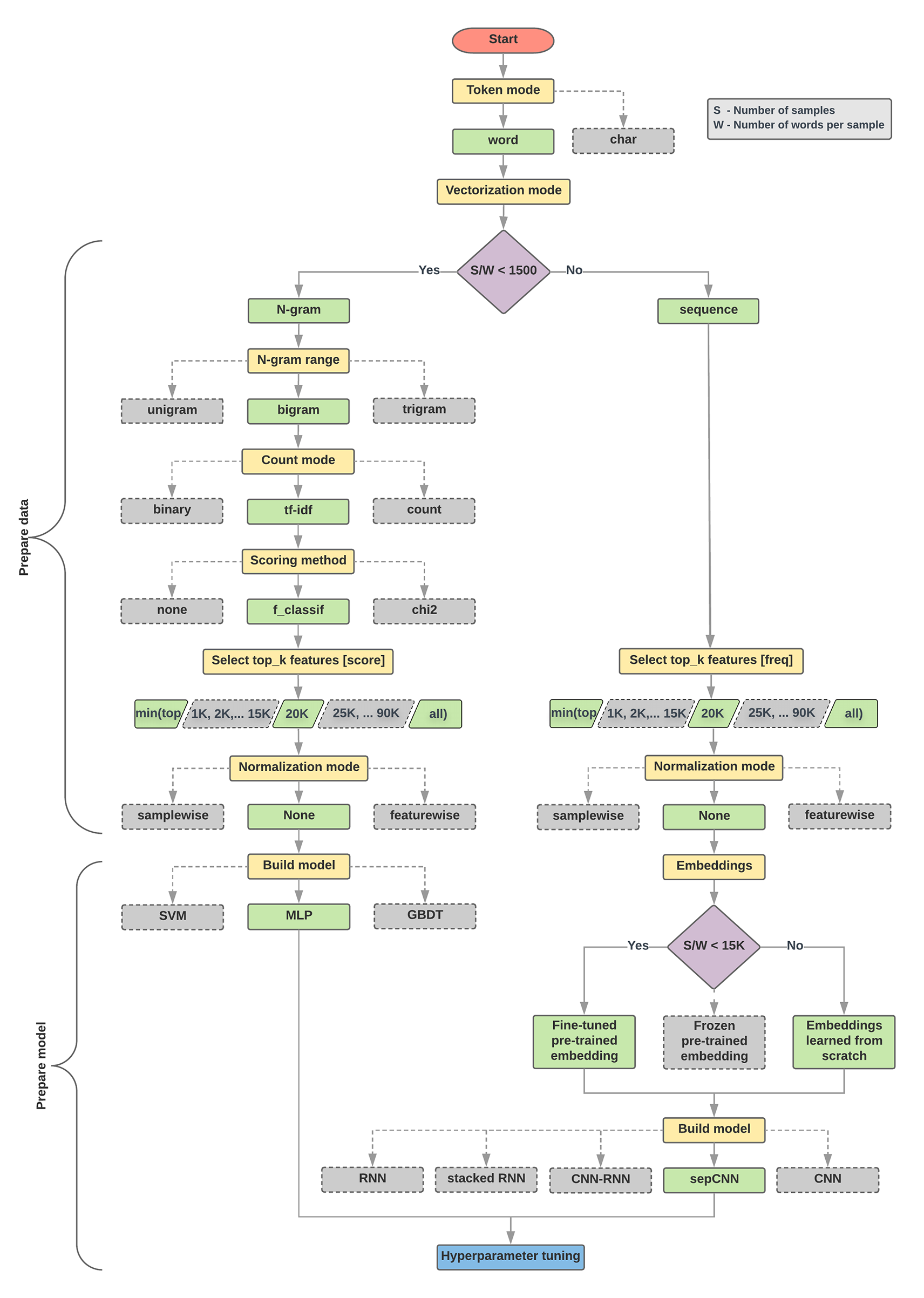# Step 2.5: Choose a Model

At this point, we have assembled our dataset and gained insights into the key characteristics of our data. Next, based on the metrics we gathered in Step 2, we should think about which classification model we should use. This means asking questions such as, “How do we present the text data to an algorithm that expects numeric input?” (this is called data preprocessing and vectorization), “What type of model should we use?”, “What configuration parameters should we use for our model?”, etc.

Thanks to decades of research, we have access to a large array of data preprocessing and model configuration options. However, the availability of a very large array of viable options to choose from greatly increases the complexity and the scope of the particular problem at hand. Given that the best options might not be obvious, a naive solution would be to try every possible option exhaustively, pruning some choices through intuition. However, that would be tremendously expensive.

In this guide, we attempt to significantly simplify the process of selecting a text classification model. For a given dataset, our goal is to find the algorithm that achieves close to maximum accuracy while minimizing computation time required for training. We ran a large number (~450K) of experiments across problems of different types (especially sentiment analysis and topic classification problems), using 12 datasets, alternating for each dataset between different data preprocessing techniques and different model architectures. This helped us identify dataset parameters that influence optimal choices.

The model selection algorithm and flowchart below are a summary of our experimentation. Don’t worry if you don’t understand all the terms used in them yet; the following sections of this guide will explain them in depth.

## Algorithm for Data Preparation and Model Building

```1. Calculate the number of samples/number of words per sample ratio.
2. If this ratio is less than 1500, tokenize the text as n-grams and use a
simple multi-layer perceptron (MLP) model to classify them (left branch in the
flowchart below):
a. Split the samples into word n-grams; convert the n-grams into vectors.
b. Score the importance of the vectors and then select the top 20K using the scores.
c. Build an MLP model.
3. If the ratio is greater than 1500, tokenize the text as sequences and use a
sepCNN model to classify them (right branch in the flowchart below):
a. Split the samples into words; select the top 20K words based on their frequency.
b. Convert the samples into word sequence vectors.
c. If the original number of samples/number of words per sample ratio is less
than 15K, using a fine-tuned pre-trained embedding with the sepCNN
model will likely provide the best results.
4. Measure the model performance with different hyperparameter values to find
the best model configuration for the dataset.
```

In the flowchart below, the yellow boxes indicate data and model preparation processes. Grey boxes and green boxes indicate choices we considered for each process. Green boxes indicate our recommended choice for each process.

You can use this flowchart as a starting point to construct your first experiment, as it will give you good accuracy at low computation costs. You can then continue to improve on your initial model over the subsequent iterations.Figure 5: Text classification flowchart

This flowchart answers two key questions:

1. Which learning algorithm or model should we use?

2. How should we prepare the data to efficiently learn the relationship between text and label?

The answer to the second question depends on the answer to the first question; the way we preprocess data to be fed into a model will depend on what model we choose. Models can be broadly classified into two categories: those that use word ordering information (sequence models), and ones that just see text as “bags” (sets) of words (n-gram models). Types of sequence models include convolutional neural networks (CNNs), recurrent neural networks (RNNs), and their variations. Types of n-gram models include logistic regression, simple multi- layer perceptrons (MLPs, or fully-connected neural networks), gradient boosted trees and support vector machines.

From our experiments, we have observed that the ratio of “number of samples” (S) to “number of words per sample” (W) correlates with which model performs well.

When the value for this ratio is small (<1500), small multi-layer perceptrons that take n-grams as input (which we'll call Option A) perform better or at least as well as sequence models. MLPs are simple to define and understand, and they take much less compute time than sequence models. When the value for this ratio is large (>= 1500), use a sequence model (Option B). In the steps that follow, you can skip to the relevant subsections (labeled A or B) for the model type you chose based on the samples/words-per-sample ratio.

In the case of our IMDb review dataset, the samples/words-per-sample ratio is ~144. This means that we will create a MLP model.

[{ "type": "thumb-down", "id": "missingTheInformationINeed", "label":"Missing the information I need" },{ "type": "thumb-down", "id": "tooComplicatedTooManySteps", "label":"Too complicated / too many steps" },{ "type": "thumb-down", "id": "outOfDate", "label":"Out of date" },{ "type": "thumb-down", "id": "samplesCodeIssue", "label":"Samples/Code issue" },{ "type": "thumb-down", "id": "otherDown", "label":"Other" }]
[{ "type": "thumb-up", "id": "easyToUnderstand", "label":"Easy to understand" },{ "type": "thumb-up", "id": "solvedMyProblem", "label":"Solved my problem" },{ "type": "thumb-up", "id": "otherUp", "label":"Other" }]